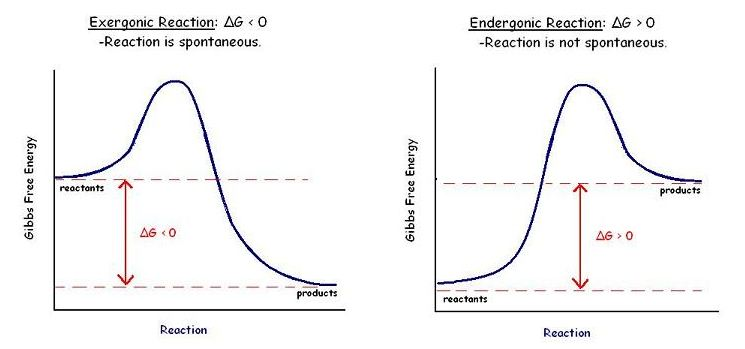# Let me free

Chemistry Level 2The Gibbs free energy, originally called available energy, was developed in the 1870's by the American mathematician Josiah Willard Gibbs. Which of following is a correct explanation about the Gibbs free energy?

(a) The Gibbs free energy always decreases in an exothermic reaction.

(b) The Gibbs free energy always increases in an endothermic reaction.

(c) In an exothermic reaction, if the reaction increases the entropy of system, then the Gibbs free energy always decreases.

(d) In an endothermic reaction, if the reaction increases the entropy of system, then the Gibbs free energy always decreases.

×